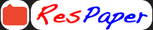Trending ▼   ResFinder# ISC Class XII Prelims 2022 : Computer Science (Father Leblond School, Darjeeling)

10 pages, 52 questions, 52 questions with responses, 54 total responses,20Koustav Chakraborty Baba Siddhnath College Of Education, Muthra BACHELOR OF EDUCATION COMPUTER SCIENCE AND APPLICATIONS
+Fave Message
 Home > rudra199140 >   F Also featured on: School Page iscFormatting page ...

FATHER LEBLOND SCHOOL SESSION 2021-22 HALF YEARLY EXAMINATION CLASS XII SUBJECT COMPUTER SCIENCE F.M 70 TIME 1hour 30 min All questions are in MCQ format. Students need to attend all the questions. Students need to show the rough work parallel to the answers (whenever required) in the answer script. Students need to write the answers in the separate answer script. The time allotted in the question paper is only writing time. Student will get separate 15 minutes to read the question paper. 1 The law that represents the Boolean equation A.A = A a) Associative b) Commutative c) Assertive d) Idempotance  2. The duality of the Boolean equation (P+1).(Q . 0) = 1 is a) (P . 0) + (Q + 1) = 0 b) (P . 0) + (Q + 1) = 0 c) (P, 1) + (Q + 0) = 0 d) None of the above  3. If A=1, B=0, C=0 and D=1, then the max term will be: a) AB C D b) A BC D c) A+B +C +D d) A +B+C+D  4. Identify the compliment of A.B + A + A .B + B a) (A +B) . A . (A+B ) . B b) (A+B) .A. (A+B) . B c) Both a and b d) (A +B) . A . (A+B ) . B  5. Identify the equivalent Boolean law for the proposition a b a) a + b b) (a + b) c) a+b d) a + b  KCH/XII/HALF THIS PAPER COMPRISES OF 10 PRINTED PAGES TURN OVERFormatting page ...

Top Contributors
to this ResPaperDhruv Ladani(29)Aman Dhol(14)Navya T G(11)Aatman Jain(1)Formatting page ...Formatting page ...Formatting page ...Formatting page ...Formatting page ...Formatting page ...Formatting page ...Formatting page ...Additional Info : ISC Class XII Sample / Model Paper 2021 : Computer Science
Tags : ISC Class XII Sample / Model Paper 2021 : Computer Science,